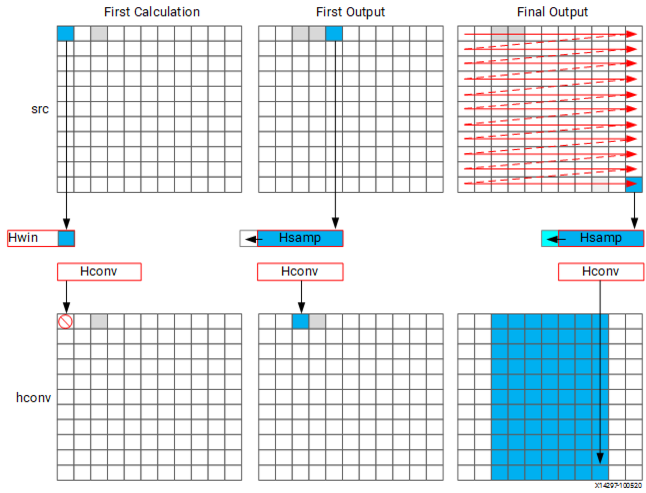# Horizontal Convolution - 2021.2 English

## Vitis High-Level Synthesis User Guide (UG1399)

Document ID
UG1399
Release Date
2021-12-15
Version
2021.2 English

To perform the calculation in a more efficient manner for FPGA implementation, the horizontal convolution is computed as shown in the following figure.

Figure 1. Streaming Horizontal ConvolutionUsing an `hls::stream` enforces the good algorithm practice of forcing you to start by reading the first sample first, as opposed to performing a random access into data. The algorithm must use the K previous samples to compute the convolution result, it therefore copies the sample into a temporary cache `hwin`. For the first calculation there are not enough values in `hwin` to compute a result, so no output values are written.

The algorithm keeps reading input samples a caching them into `hwin`. Each time it reads a new sample, it pushes an unneeded sample out of `hwin`. The first time an output value can be written is after the Kth input has been read. Now an output value can be written.

The algorithm proceeds in this manner along the rows until the final sample has been read. At that point, only the last K samples are stored in `hwin`: all that is required to compute the convolution.

The code to perform these operations is shown below.

``````// Horizontal convolution
HConvW:for(int row = 0; row < width; row++) {
HconvW:for(int row = border_width; row < width - border_width; row++){
T out_val = 0;
HConv:for(int i = 0; i < K; i++) {
hwin[i] = i < K - 1 ? hwin[i + 1] : in_val;
out_val += hwin[i] * hcoeff[i];
}
if (row >= K - 1)
hconv << out_val;
}
}
``````

An interesting point to note in the code above is use of the temporary variable `out_val` to perform the convolution calculation. This variable is set to zero before the calculation is performed, negating the need to spend 2 million clocks cycle to reset the values, as in the previous example.

Throughout the entire process, the samples in the `src` input are processed in a raster-streaming manner. Every sample is read in turn. The outputs from the task are either discarded or used, but the task keeps constantly computing. This represents a difference from code written to perform on a CPU.

In a CPU architecture, conditional or branch operations are often avoided. When the program needs to branch it loses any instructions stored in the CPU fetch pipeline. In an FPGA architecture, a separate path already exists in the hardware for each conditional branch and there is no performance penalty associated with branching inside a pipelined task. It is simply a case of selecting which branch to use.

The outputs are stored in the `hls::stream hconv` for use by the vertical convolution loop.